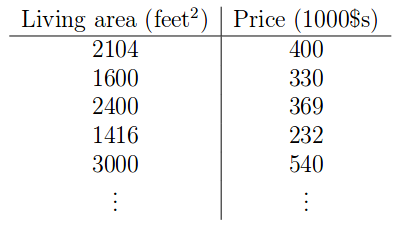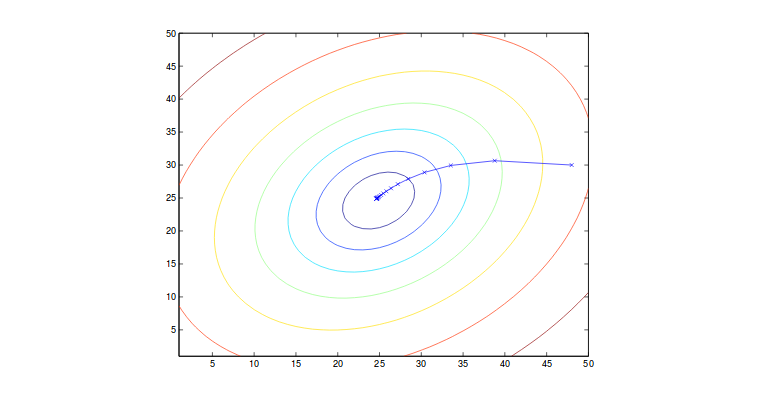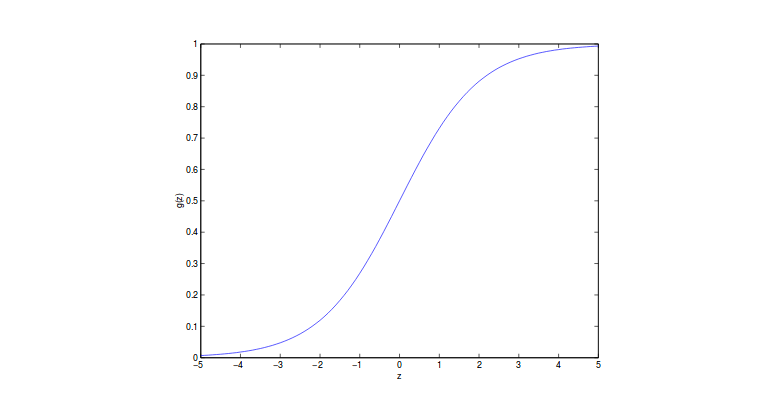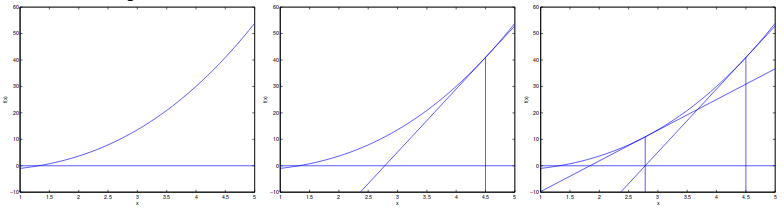# Discriminative Algorithm Chinese Version# 1 线性回归 Linear Regression

## 最小均方算法Least Mean Square(LMS) algorithm

LMS算法主要使用梯度下降(gradient descent)来找到局部最小值(local minimum)。 为了实现它，我们将参数初始化为0，即 $\theta = \overrightarrow{0}$ ，然后用以下方法来反复更新 $\theta$：# 5 分类与逻辑回归# 7 牛顿最大化方法# 8 广义线性模型和指数族

T，a和b是固定参数，我们可以通过改变 $\eta$ 来建立不同的分布。 现在，我们可以证伯努利分布和高斯分布属于指数族:

# 9 构建广义线性模型

(1)我们总是假设 $y \lvert x;\theta \thicksim \text{ExponentialFamily}(\eta)$.

(2)通常，我们想要预测给定x的T（y）的期望值。 最有可能的是，我们有 $T(y) = y$。定义上，我们有 $h(x) = \mathbb{E}[y\lvert x]$，这对于逻辑回归和线性回归都是正确的。 注意，在逻辑回归中，我们总是有 $\mathbb{E}[y\lvert x] = p(y=1\lvert x;\theta)$

(3)输入和自然参数关联为：$\eta = \theta^Tx$

## 9.2 逻辑回归

。 通常，响应函数是 $\eta$ 的函数，并给出 $\eta$ 和分布参数之间的关系，而链接函数产生η作为分布参数的函数。 反函数意味着用一者来表达另一者。 从上面的推导，我们知道伯努利的响应函数是逻辑函数，而高斯函数的典型响应函数是均值函数。

## 9.3 Softmax回归

$a(\eta) = -\log(\phi_k)$ and $b(y) = 1$

Tags: